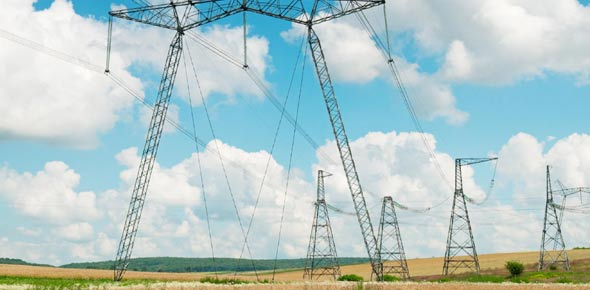# Voltage And Current Divider

11 QuestionsSettingsMaterial on Ohm's Law, Voltage, Current, and Resistance.

Related Topics
• 1.
What is the unit for current?
• A.

Volts

• B.

Amperes

• C.

Ohms

• D.

Joules

• 2.
What is shown in this picture?
• A.

Resistors connected in parallel

• B.

Batteries connected in parallel

• C.

Resistors connected in series

• D.

Batteries connected in series

• 3.
What is current?
• A.

The flow of charges, usually in the form of electrons

• B.

The newness of a battery or resistor

• C.

Something that blocks or slows the flow of electrons

• D.

The potential energy stored in a battery

• 4.
What is the unit for voltage?
• A.

Volts

• B.

Amperes

• C.

Ohms

• D.

Joules

• 5.
• A.

I1=8A, I2=6A

• B.

I1=1A, I2=3A

• C.

I1=8.3A, I2=1.6A

• D.

None of them

• 6.
What is the unit for resistance?
• A.

Volts

• B.

Amperes

• C.

Ohms

• D.

Joules

• 7.
Which of these is the equation for current divider?
• A.

I2= (R1/R1+R2) * l

• B.

V = IR

• C.

R = IV

• D.

PE = mgh

• 8.
In the picture, how resistance is connacted?
• A.

In series

• B.

In parallel

• C.

They are not connected

• 9.
In the picture, calculate R2 and R3 to be one resistance,also the voltage of R23?
• A.

17.5kohm , 3,5V

• B.

17.5kohm , 7,5V

• C.

None of them

• 10.
How can measurment the voltage divider in the circuit?
• A.

Series

• B.

Parallel

• C.

None of them

• 11.
In this circuit diagram, what are R1, R2, and R3?
• A.

Batteries connected in series

• B.

Batteries connected in parallel

• C.

Resistors connected in series

• D.

Resistors connected in parallel